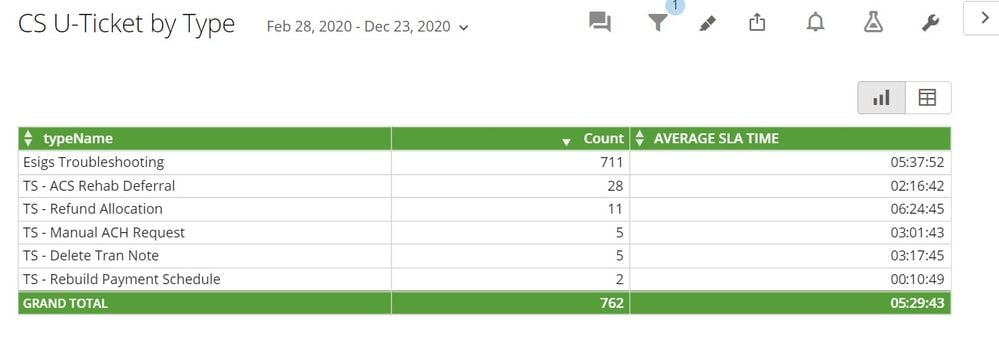# % of Total

Hi,

I am currently working with a dataset with text values that I want to count and get the percent total.

I can do the count since Domo allows you to count text values, but I can't do the % total. I wanted to include another column that would give the sum of the count, then I would be able to do the % total.

I tried SUM(COUNT('typename') as well as 'typename'/ COUNT('typename') and none of those gave me the results I need.•CoachYou'll want to utilize a windowing function to calculate the sum for each partition / typename and then divide that by the sum of the entire dataset.

`SUM(SUM(1)) OVER (PARTITION BY `typename`) / SUM(SUM(1)) OVER ()`

If you don't have windowing functions enabled you'll need to talk with your CSM to get that feature switched on.

**Did this solve your problem? Accept it as a solution!**

•CoachYou'll want to utilize a windowing function to calculate the sum for each partition / typename and then divide that by the sum of the entire dataset.

`SUM(SUM(1)) OVER (PARTITION BY `typename`) / SUM(SUM(1)) OVER ()`

If you don't have windowing functions enabled you'll need to talk with your CSM to get that feature switched on.